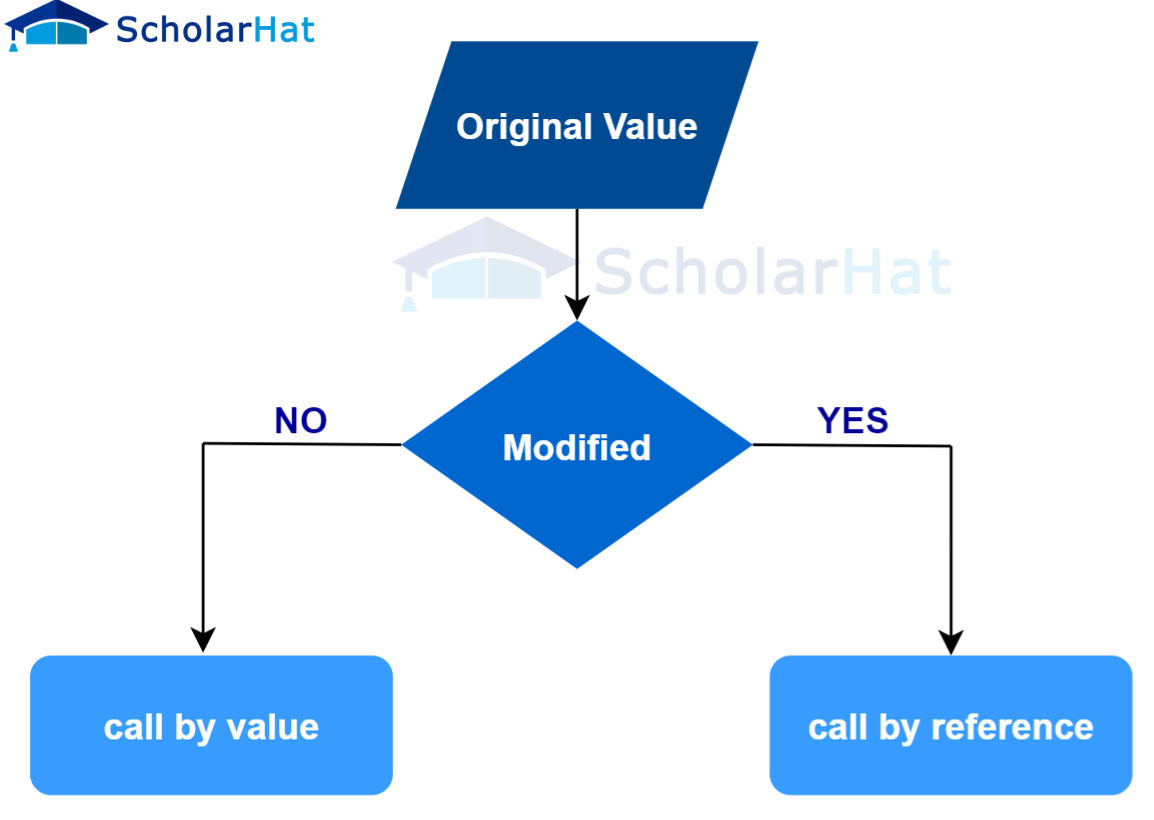# Call by Value and Call by Reference in C

24 Nov 2023
Intermediate
409 ViewsLearn via Video Course & by Doing Hands-on Labs

## Call by Value and Call by Reference in C: An Overview

Have you ever wondered what the difference is between method calls made using call by value and call by reference? If so, you have come to the right place. In this C tutorial, we will be looking at `call by value` and `call by reference` in C. Our C training resources will also help you gain hands-on experience and practical knowledge. After reading this article, not only you will have a good understanding of both techniques but also feel confident enough to apply them wherever necessary.

## Call by Value and Call by Reference in C

We have already seen `functions` and their various aspects like `formal parameters` and `actual parameters` in the section, Functions in C. In the `function call`, we used to pass `parameters/arguments/data` to the function which is being called.

In this section, we will look at the two ways in which `parameters` are passed in the `function call` in the C language.## Call by Value in C

In this method, the value of the `actual parameter` is passed to the corresponding `formal parameter` of the called function. This technique ensures that the original data i.e. `actual parameter` does not get altered by the called function, as the called function deals only with a copy of that data.

When a programmer opts for the `call by value`, the memory space for the `formal parameters` is allocated separately in the `function stack`, which further reinforces the fact that the original data remains untouched. Thus, `formal parameters` and `actual parameters` are allocated to different memory locations.

Example

``````
#include <stdio.h>
void swap(int x, int y) {
int temp = x;
x = y;
y = temp;
}
int main() {
int a = 40, b = 50;
printf("Before swap, a = %d and b = %d\n", a, b);
swap(a, b);
printf("After swap, a = %d and b = %d\n", a, b);
return 0;
}``````

The above program swaps the values of the variables, `a` and `b`. In the `main()` function, the `function call` passes the `actual parameter` values i.e. values of `a` and `b` to the `swap()` function. The `swap()` function swaps the values of `a` and `b`.

Due to the `call-by-value` method, the value of original variables `a` and `b` remains unchanged even after swapping by the `swap()` function.

#### Output

``````Before swap, a = 40 and b = 50
After swap, a = 40 and b = 50``````

## Call by Reference in C

In this method, instead of passing the values of the `actual parameters` to the formal ones, the addresses of the `actual parameters` are passed. Therefore, the modification by the called function to the `formal parameters` affects the `actual parameters` as well.

When a programmer opts for the `call by reference`, the memory space for the `formal parameters` and the `actual parameters` are the same. All the operations in the function are performed on the value stored at the address of the `actual parameters`, and the modified value gets stored at the same address.

This technique not only conserves memory but also simplifies collaboration, as complex data types like `arrays` or `structures` can easily be manipulated without being duplicated needlessly.

#### Example

``````
#include <stdio.h>
void swap(int *a, int *b) {
int temp = *a;
*a = *b;
*b = temp;
}
int main() {
int x = 5;
int y = 10;
printf("Before swap: x = %d, y = %d\n", x, y);
swap(&x, &y);
printf("After swap: x = %d, y = %d\n", x, y);
return 0;
}``````

#### Output

``````Before swap: x = 5, y = 10
After swap: x = 10, y = 5``````

## Differences between call by value and call by reference in C

 Properties Call by value Call by reference Data copying In call by value, a copy of the value of the argument is passed to the function In call by reference, the memory address of the argument is passed to the function. Original value modification any changes made to the parameter inside the function do not affect the original value passed to the function any changes made to the parameter inside the function affect the original value passed to the function. Memory usage Call by value needs more memory Call by reference uses less memory Performance Call by value is generally faster than call by reference Call by reference is slower than call by value Function definition In call by value, the function definition does not require any special syntax In call by reference, the parameter must be declared as a C language pointer in the function definition.
##### Summary

`call by value` and `call by reference` in C are important concepts to understand if you want a deeper conceptual understanding of computer programming. `Call by value` copies a variable's value whereas `call by reference` passes a `pointer` instead. Depending on what the output is supposed to be, developers need to decide which one they should use.

Consider enhancing your knowledge and gaining recognition by enrolling in C Certification Training, which will provide comprehensive guidance and hands-on experience in mastering various concepts and techniques in C programming.

Share Article
Similar Articles